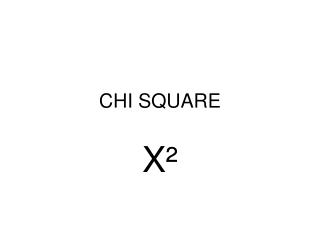DownloadDownload PresentationCHI SQUARE

# CHI SQUARE

Télécharger la présentation## CHI SQUARE

- - - - - - - - - - - - - - - - - - - - - - - - - - - E N D - - - - - - - - - - - - - - - - - - - - - - - - - - -
##### Presentation Transcript

1. “MATHEMATICAL JUDGE” OF PROBABILITIES • X² =∑{(O-E)²/E} • ∑= the sum of • O= observed E= expected

2. Chi square is used to measure the significance of the data in comparison with what you expect to get.

3. Chi square table

4. Problem #1 • F2= 5,474 yellow seeds & 1850 green seeds • 1. Find the total # of seeds • 2. What is the expected ratio? • 3. How may yellow and green seeds would you expect? Yellow________,green____

5. 4. For yellow seeds, used the formula • (O-E)²/E. Yellow ________ • 5. For green seeds , use above formula. green _______ 6. Add the #’s together.

6. Did you get? • 0.263 • Yea!!!!!!!!!!!!!!!!!

7. 7. Compare your chi square valve to the Chi square table.

8. With one degree of freedom we could be allowed a chi square as large as 3.84 and the results would still be considered significant to 5%. X² = 0.263 there’s less than a 5% chance this 3:1 happened by accident.

9. The degrees of freedom are one less than the number of categories you have to work with. We have two categories, yellow and green, so we have one degree of freedom.

10. Find the chi square for the C-fern. • Use the class totals. • The p= cc X Cc • cc= polka dot and is recessive • Cc = wild type and heterozygous • What would the expected ratio for F1 be with this cross?